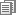Excel 2007重大bug：850×77.1＝1000002007-09-26 15:15:55  出处：快科技 作者：上方文Q 编辑：上方文Q=5.1*12850
=10.2*6425
=20.4*3212.5
=40.8*1606.25
=154.2*425
=212.5*308.4
=308.4*212.5
=425*154.2###### 文章观点支持

+0
+0